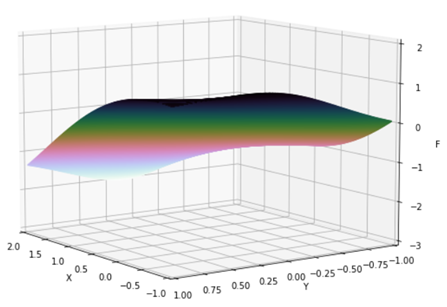top of page
Search

# Python Implementation of ADJIMAN FUNCTION

Updated: Jul 14, 2021Mathematical DefinitionInput Domain

The function is defined on input domain i.e. x ∈ [−1, 2] and y ∈ [−1, 1].

Global Minima

The global minimum f(z) = −2.02181 is located at z = (0, 0).

Characteristics

The function is not convex.

The function is differentiable.

The function is non-separable.

The function is defined on 2-dimensional space.

Python Implementation

```% Please forward any comments or bug reports in chat
Copyright 2021. INDUSMIC PRIVATE LIMITED.THERE IS NO WARRANTY, EXPRESS OR IMPLIED. WE DO NOT ASSUME ANY LIABILITY FOR THE USE OF THIS PROGRAM. If software is modified to produce derivative works, such modified software should be clearly marked. Additionally, user can redistribute it and/or modify it under the terms of the GNU General Public License. This program is distributed in the hope that it will be useful, but WITHOUT ANY WARRANTY. See the GNU General Public License for more details.
% for any support connect with us on help.indusmic@gmail.com
% Author: Yamini Jain

from mpl_toolkits import mplot3d
import matplotlib.pyplot as plt
import numpy as np
from matplotlib import cm
def f(x,y):
return (np.cos(x)*np.sin(y) - (x/(y**2+1)))
X = np.linspace(-1,2)
Y = np.linspace(-1,1)
x,y = np.meshgrid(X,Y) #creating a rough mesh for generation of graph
F = f(x,y)
fig = plt.figure(figsize=(9,9))
ax = plt.axes(projection='3d')
ax.contour3D(x,y, F,450, cmap = cm.cubehelix_r)
ax.set_title('Adijman Function') #Giving a title to the image
ax.set_xlabel('X')
ax.set_ylabel('Y')
ax.set_zlabel('F')
ax.set_xlim(2,-1)
ax.set_ylim(1,-1)
ax.set_zlim(-3,2)
ax.view_init(21,45) #This can be altered according to the need
plt.show()

```

References:

 Jamil, Momin, and Xin-She Yang. "A literature survey of benchmark functions for global optimization problems." International Journal of Mathematical Modelling and Numerical Optimization 4.2 (2013): 150-194.